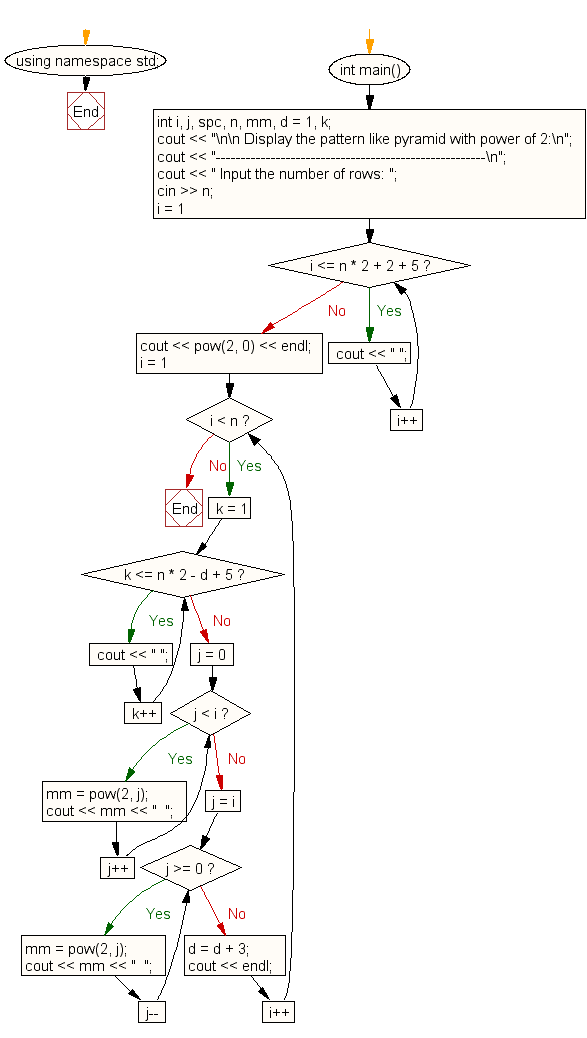﻿ C++ Exercises: Display the pattern power of 2, triangle - w3resource

# C++ Exercises: Display the pattern power of 2, triangle

## C++ For Loop: Exercise-54 with Solution

Write a program in C++ to display the pattern like pyramid, power of 2.

Sample Solution:

C++ Code :

``````#include <iostream>
#include <math.h>
using namespace std;

int main()
{
int i, j, spc, n, mm, d = 1, k;
cout << "------------------------------------------------------\n";
cout << " Input the number of rows: ";
cin >> n;
//----------- space for first line ----------------------
for (i = 1; i <= n * 2 + 2 + 5; i++) //extra 5 spaces is the margin from left
cout << " ";
cout << pow(2, 0) << endl;
for (i = 1; i < n; i++)
{
//----------- printing spaces from 2nd line to end -------
for (k = 1; k <= n * 2 - d + 5; k++)
{
cout << " ";
}
//----------- print upto middle ----------------
for (j = 0; j < i; j++)
{
mm = pow(2, j);
cout << mm << "  "; //print 2 spaces
}
//------------- print after middle to end -------
for (j = i; j >= 0; j--)
{
mm = pow(2, j);
cout << mm << "  "; //print 2 spaces
}
d = d + 3;
cout << endl;
}
}

``````

Sample Output:

```Input the number of rows: 5
1
1  2  1
1  2  4  2  1
1  2  4  8  4  2  1
1	2  4  8  16  8  4  2  1

```

Flowchart:C++ Code Editor: